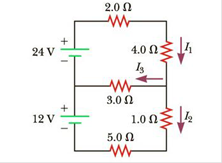Chapter 18, Problem 27P

Chapter
Section
Textbook Problem

(a) Can the circuit shown in Figure P18.27 be reduced to a single resistor connected to the batteries? Explain. (b) Calculate each of the unknown currents I1, I2, and I3 for the circuit.Figure P18.27

(a)

To determine
To reduce the circuits to a single resistor connected to the batteries.

Explanation

Given Info: Resistance R1 is 2.0Ω , Resistance R2 is 4.0Ω , Resistance R3 is 3.0Ω Resistance R4 is 1.0Ω , Resistance R5 is 5.0Ω .

Explanation:

Some simplification can be made to reduce the circuit.

Formula to calculate to reduce to a single resistor is by equivalent resistance REq upper in series

From the Upper loop:

Two resistances are connected in series R1 and R2

REq upper=R1+R2 (I)

Formula to calculate to reduce to a single resistor is by equivalent resistance REq lower in series

From the lower loop:

Two resistances are connected in series R4 and R5

REq lower=R4+R5 (II)

• REq upper is Equivalent resistance in the upper loop
• REq lower is Equivalent resistance in the lower loop
• R1,R2,R3,R4,R5 is corresponding resistance in the circuits
• I1,I2,I3 is corresponding current in the circuits

Substitute 2

(b)

To determine
The current from the circuits I1,I2,I3 .

Still sussing out bartleby?

Check out a sample textbook solution.

See a sample solution

The Solution to Your Study Problems

Bartleby provides explanations to thousands of textbook problems written by our experts, many with advanced degrees!

Get Started